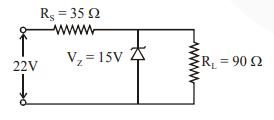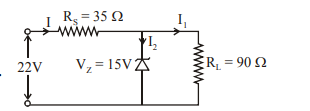# The value of power dissipated across the zener diodeQuestion:

The value of power dissipated across the zener diode $\left(\mathrm{V}_{\mathrm{z}}=15 \mathrm{~V}\right)$ connected in the circuit as shown in the figure is $x \times 10^{-1}$ watt.The value of x, to the nearest integer, is ______.

Solution:Voltage across $R_{S}=22-15=7 V$

Current through $\mathrm{R}_{\mathrm{S}}=\mathrm{I}=\frac{7}{35}=\frac{1}{5} \mathrm{~A}$

Current through $90 \Omega=\mathrm{I}_{2}=\frac{15}{90}=\frac{1}{6} \mathrm{~A}$

Current through zener $=\frac{1}{5}-\frac{1}{6}=\frac{1}{30} \mathrm{~A}$

Power through zener diode

$\mathrm{P}=\mathrm{VI}$

$P=15 \times \frac{1}{30}=0.5 \mathrm{watt}$

$\mathrm{P}=5 \times 10^{-1}$ watt